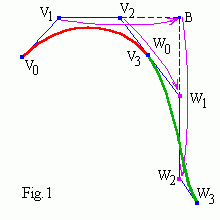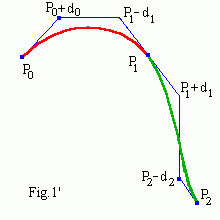# Bezier control points

Let us consider again bicubic uniform B-spline composed of n segments. We know that B-spline doesn't interpolate its deBoor control points. As since Bezier curve goes through its terminal control points we will use Bezier points now.
We have seen (look at Fig.1), that to determine cubic B-spline we need to set its (n+3) points. E.g. all control points of the first Bezier curve and one independent "last" point for each new segment. But it is more natural to set tangents at terminal points of the B-spline (that is we will use V1 and W2 points instead of V1 and V2 in Fig.1). Fig.1' shows, that all Bezier control points of the curve are evaluated from two vectors (P0 , P1 , ... , Pn) and (d0 , d1 , ... , dn). Points (P0 , P1 , ... , Pn), d0 , dn are fixed and d1 , d2 , ... , dn-1 should be calculated.
Interpolating B-spline applet (the right window). Drag the mouse to move the nearest control point (a small blue square). Click mouse + "Shift"("Ctrl") to add (remove) a point.# Solving banded equations

It is evident that by definition the curve is C1 continuous. From C2 continuity it follows, e.g.
P1 - 2(P1 - d1) + (P0 + d0) = (P2 - d2) - 2(P1 + d1) + P1 ,
d0 + 4d1 + d2 = P2 - P0 .

In general case we get banded 3-diagonals linear equations
4d1 + d2 = P2 - P0 - d0
d1 + 4d2 + d3 = P3 - P1
... ...
di + 4di+1 + di+2 = Pi+2 - Pi
... ...
dn-2 + 4dn-1 = Pn - Pn-2 - dn

We can successively express
di = Ai + Bi di+1 ,     i = 1 , 2 , ... , n-1
where
A1 = (P2 - P0 - d0)/4 ,     B2 = -1/4,
Ai = (Pi+1 - Pi-1 - Ai-1)/(4 + Bi-1) ,     Bi = -1/(4 + Bi-1) .

Then we will find dn-1 and di successively by iterations in reverse order
dn-1 = An-1 + Bn-1 dn ,     di = ... .

It is programmed as (Bint.java)

```public void findCPoints(){
Bi = -.25;
Ax = (Px - Px - dx)/4;   Ay = (Py - Py - dy)/4;
for (int i = 2; i < N-1; i++){
Bi[i] = -1/(4 + Bi[i-1]);
Ax[i] = -(Px[i+1] - Px[i-1] - Ax[i-1])*Bi[i];
Ay[i] = -(Py[i+1] - Py[i-1] - Ay[i-1])*Bi[i]; }
for (int i = N-2; i > 0; i--){
dx[i] = Ax[i] + dx[i+1]*Bi[i];  dy[i] = Ay[i] + dy[i+1]*Bi[i]; }
}
```
You can compare here Interpolating B-spline and Cardinal curve.

# Handling the terminal tangents

If you don't like to worry about terminal tangents of interpolating curve, you can direct them to the nearest point as for Cardinal curve, i.e. ("1/3 rule" is used)
d0 = (P1 - P0)/3,     dn = (Pn - Pn-1)/3 .

Interpolating applet without terminal tangents. Drag the mouse to move a control point. Click mouse + "Shift"("Ctrl") to add (remove) a point.

We don't consider here closed curves, nonuniform splines and node insertion.

Don't you know the name of the interpolating B-spline?

Contents   Previous: Nonuniform B-splines   Next: NURBS
updated 21 August 2001